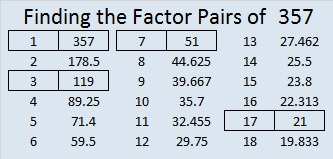# 357 Equality, Mathematics, and Dr. Martin Luther King, Jr.

I read several quotes from Dr. Martin Luther Kings, Jr. today, including this one on equality and integration. The last sentence mentions mathematics, but I’m not exactly sure what that last sentence has to do with the rest of the quotation. Can anyone enlighten me? (Check the comments for one interpretation.)Here is a little about the number 357:

3, 5, and 7 are three consecutive odd numbers so, so 357 is divisible by 3 and is a composite number.

• 357 is a composite number.
• Prime factorization: 357 = 3 x 7 x 17
• The exponents in the prime factorization are 1, 1, and 1. Adding one to each and multiplying we get (1 + 1)(1 + 1)(1 + 1) = 2 x 2 x 2 = 8. Therefore 357 has exactly 8 factors.
• Factors of 357: 1, 3, 7, 17, 21, 51, 119, 357
• Factor pairs: 357 = 1 x 357, 3 x 119, 7 x 51, or 17 x 21
• 357 has no square factors that allow its square root to be simplified. √357 ≈ 18.894